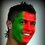# Integration

$\large \int \dfrac{\arctan(x)}{x} \, dx$ Can anyone tell how this integration can be done?Note by Pratik Roy
5 years ago

This discussion board is a place to discuss our Daily Challenges and the math and science related to those challenges. Explanations are more than just a solution — they should explain the steps and thinking strategies that you used to obtain the solution. Comments should further the discussion of math and science.

When posting on Brilliant:

• Use the emojis to react to an explanation, whether you're congratulating a job well done , or just really confused .
• Ask specific questions about the challenge or the steps in somebody's explanation. Well-posed questions can add a lot to the discussion, but posting "I don't understand!" doesn't help anyone.
• Try to contribute something new to the discussion, whether it is an extension, generalization or other idea related to the challenge.
• Stay on topic — we're all here to learn more about math and science, not to hear about your favorite get-rich-quick scheme or current world events.

MarkdownAppears as
*italics* or _italics_ italics
**bold** or __bold__ bold
- bulleted- list
• bulleted
• list
1. numbered2. list
1. numbered
2. list
Note: you must add a full line of space before and after lists for them to show up correctly
paragraph 1paragraph 2

paragraph 1

paragraph 2

[example link](https://brilliant.org)example link
> This is a quote
This is a quote
    # I indented these lines
# 4 spaces, and now they show
# up as a code block.

print "hello world"
# I indented these lines
# 4 spaces, and now they show
# up as a code block.

print "hello world"
MathAppears as
Remember to wrap math in $$ ... $$ or $ ... $ to ensure proper formatting.
2 \times 3 $2 \times 3$
2^{34} $2^{34}$
a_{i-1} $a_{i-1}$
\frac{2}{3} $\frac{2}{3}$
\sqrt{2} $\sqrt{2}$
\sum_{i=1}^3 $\sum_{i=1}^3$
\sin \theta $\sin \theta$
\boxed{123} $\boxed{123}$

## Comments

Sort by:

Top Newest

you need knowledge of euler's formula and polylogarithm. first we write $\tan(y)=x\to x=\arctan(x)=y$ or $x=\dfrac{\sin(y)}{\cos(y)}=-i\dfrac{e^{2iy}-1}{e^{2iy}+1}$ So $y=\dfrac{i}{2} \ln(1+ix)-\dfrac{i}{2} \ln(1-ix)$ so, $\arctan(x)=\dfrac{i}{2} \ln(1+ix)-\dfrac{i}{2} \ln(1-ix)$ putting this in the integral $I=\dfrac{i}{2}\int \dfrac{ \ln(1+ix)-\ln(1-ix)}{x}dx= \dfrac{i}{2}(li_2(-ix)-li_2(ix))+C$

- 5 years ago

Log in to reply

can you please suggest me from where i could learn more about doing these type of integrations.

- 5 years ago

Log in to reply

from here try the integration tricks wikis and advance integration. while this is not complete yet the contents there are good enough for this problem.

- 5 years ago

Log in to reply

thanks

- 5 years ago

Log in to reply

how can i calculate the integral if the limits are given?

- 5 years ago

Log in to reply

×

Problem Loading...

Note Loading...

Set Loading...